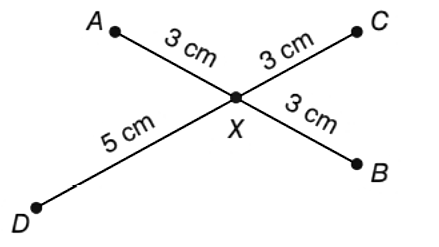Chapter 1.2, Problem 33E### Elementary Geometry for College St...

6th Edition
Daniel C. Alexander + 1 other
ISBN: 9781285195698

#### Solutions

Chapter
Section### Elementary Geometry for College St...

6th Edition
Daniel C. Alexander + 1 other
ISBN: 9781285195698
Textbook Problem
1 views

# A line segment is bisected if its two parts have the same length. Which line segment, A B ¯ or C D ¯ , is bisected at point X ?To determine

To find:

Which of the line segment is bisected.

Explanation

Calculation:

Given,

From the above figure,

A×=3 cm,

B×=3 cm,

C×=3 cm, and

D×=5 cm

### Still sussing out bartleby?

Check out a sample textbook solution.

See a sample solution

#### The Solution to Your Study Problems

Bartleby provides explanations to thousands of textbook problems written by our experts, many with advanced degrees!

Get Started

#### In problems 45-62, perform the indicated operations and simplify. 54.

Mathematical Applications for the Management, Life, and Social Sciences

#### solve the equation by using the quadratic formula. 136. 3x2 4x + 1 = 0

Applied Calculus for the Managerial, Life, and Social Sciences: A Brief Approach

#### The graph of is: a) b) c) d)

Study Guide for Stewart's Multivariable Calculus, 8th

#### limx0cscx= a) 0 b) c) d) does not exist

Study Guide for Stewart's Single Variable Calculus: Early Transcendentals, 8th